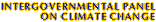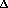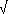Climate Change 2001: Working Group I: The Scientific BasisGet Javascript Other reports in this collection

### 6.3.4 Total Well-Mixed Greenhouse Gas Forcing Estimate

The radiative forcing due to all well-mixed greenhouse gases since pre-industrial times was estimated to be 2.45 Wm-2 in the SAR with an uncertainty of 15%. This is now altered to a radiative forcing of 2.43 Wm-2 with an uncertainty of 10%, based on the range of model results and the discussion of factors leading to uncertainties in the radiative forcing due to these greenhouse gases. The uncertainty in the radiative forcing due to CO2 is estimated to be smaller than for the other well-mixed greenhouse gases; less than 10% (Section 6.3.1). For the CH4 forcing the main uncertainty is connected to the radiative transfer code itself and is estimated to be about 15% (Section 6.3.2). The uncertainty in N2O (Section 6.3.2) is similar to that for CO2, whereas the main uncertainties for halocarbons arise from the spectroscopic data. The estimated uncertainty for halocarbons is 10 to 15% for the most frequently studied species, but higher for some of the less investigated molecules (Section 6.3.3). A small increase in the concentrations of the well-mixed greenhouse gases since the SAR has compensated for the reduction in radiative forcing resulting from improved radiative transfer calculations. The rate of increase in the well-mixed greenhouse gas concentrations, and thereby the radiative forcing, has been smaller over the first half of the 1990s compared to previous decades (see also Hansen et al., 1998). This is mainly a result of reduced growth in CO2 and CH4 concentrations and smaller increase or even reduction in the concentration of some of the halocarbons.

### 6.3.5 Simplified Expressions

IPCC (1990) used simplified analytical expressions for the well-mixed greenhouse gases based in part on Hansen et al. (1988). With updates of the radiative forcing, the simplified expressions need to be reconsidered, especially for CO2 and N2O. Shi (1992) investigated simplified expressions for the well-mixed greenhouse gases and Hansen et al. (1988, 1998) presented a simplified expression for CO2. Myhre et al. (1998b) used the previous IPCC expressions with new constants, finding good agreement (within 5%) with high spectral resolution radiative transfer calculations. The already well established and simple functional forms of the expressions used in IPCC (1990), and their excellent agreement with explicit radiative transfer calculations, are strong bases for their continued usage, albeit with revised values of the constants, as listed in Table 6.2. Shi (1992) has suggested more physically based and accurate expressions which account for (i) additional absorption bands that could yield a separate functional form besides the one in IPCC (1990), and (ii) a better treatment of the overlap between gases. WMO (1999) used a simplified expression for CO2 based on Hansen et al. (1988) and this simplified expression is used in the calculations of GWP in Section 6.12. For CO2 the simplified expressions from Shi (1992) and Hansen et al. (1988) are also listed alongside the IPCC (1990)-like expression for CO2 in Table 6.2. Compared to IPCC (1990) and the SAR and for similar changes in the concentrations of well-mixed greenhouse gases, the improved simplified expressions result in a 15% decrease in the estimate of the radiative forcing by CO2 (first row in Table 6.2), a 15% decrease in the case of N2O, an increase of 10 to 15% in the case of CFC-11 and CFC-12, and no change in the case of CH4.

 Table 6.2: Simplified expressions for calculation of radiative forcing due to CO2, CH4, N2O, and halocarbons. The first row for CO2 lists an expression with a form similar to IPCC (1990) but with newer values of the constants. The second row for CO2 is a more complete and updated expression similar in form to that of Shi (1992). The third row expression for CO2 is from WMO (1999), based in turn on Hansen et al. (1988).Trace gas Simplified expression Radiative forcing,F (Wm-2) ConstantsCO2F =ln(C/C0)= 5.35F=ln(C/C0) + ß (C -C0)= 4.841, ß = 0.0906F=(g(C)g(C0)) where g(C)= ln(1+1.2C+0.005C2 +1.4 x 10-6C3)= 3.35CH4F=(M M0)  (f(M,N0)  f(M0,N0))= 0.036N2OF=(N N0)  (f(M0,N)  f(M0,N0))= 0.12CFC-11aF=(X  X0)= 0.25CFC-12F=(X  X0)= 0.32f(M,N) = 0.47 ln[1+2.01x10-5 (MN)0.75+5.31x10-15 M(MN)1.52] C is CO2 in ppm M is CH4 in ppb N is N2O in ppb X is CFC in ppb The constant in the simplified expression for CO2 for the first row is based on radiative transfer calculations with three-dimensional climatological meteorological input data (Myhre et al., 1998b). For the second and third rows, constants are derived with radiative transfer calculations using one-dimensional global average meteorological input data from Shi (1992) and Hansen et al. (1988), respectively. The subscript 0 denotes the unperturbed concentration. a The same expression is used for all CFCs and CFC replacements, but with different values for(i.e., the radiative efficiencies in Table 6.7).